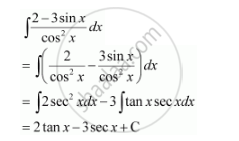Share

# Find the Following Integrals Int (2 - 3 Sinx)/(Cos^2 X) Dx - CBSE (Science) Class 12 - Mathematics

ConceptIntegration as an Inverse Process of Differentiation

#### Question

Find the following integrals int (2 - 3 sinx)/(cos^2 x) dx

#### SolutionIs there an error in this question or solution?

#### Video TutorialsVIEW ALL 

Solution Find the Following Integrals Int (2 - 3 Sinx)/(Cos^2 X) Dx Concept: Integration as an Inverse Process of Differentiation.
S